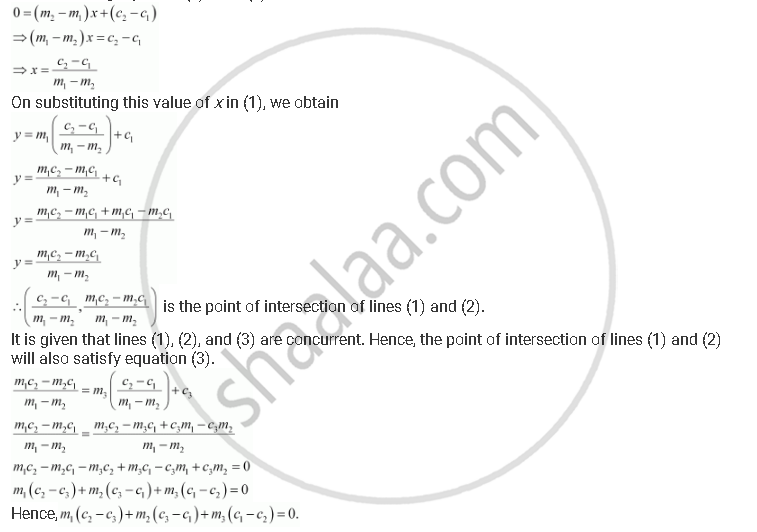# If Three Lines Whose Equations Are Y = M1x + C1, Y = M2x + C2 and Y = M3x + C3 Are Concurrent, Then Show that M1(C2 – C3) + M2 (C3 – C1) + M3 (C1 – C2) = 0. - Mathematics

If three lines whose equations are y = m1x + c1, y = m2x + c2 and y = m3x + c3 are concurrent, then show that m1(c2 – c3) + m2 (c3 – c1) + m3 (c1 – c2) = 0.

#### Solution

The equations of the given lines are

y = m1x + c1 … (1)

y = m2x + c2 … (2)

y = m3x + c3 … (3)

On subtracting equation (1) from (2), we obtainConcept: General Equation of a Line
Is there an error in this question or solution?

#### APPEARS IN

NCERT Class 11 Mathematics
Chapter 10 Straight Lines
Q 10 | Page 233